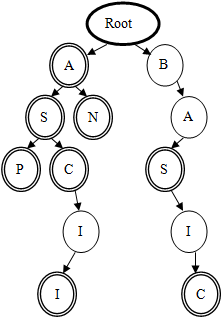## Day 2, Problem 1 - Trie

When performing grammar analyses, it is commonly needed to check whether a word is present in our word list. To improve the speed of the search, it is common to draw out the corresponding trie for the word list, which is characterized as follows:

• The root node does not contain letters, but every other node other than the root each contains a single uppercase English letter.
• For a path from the root to any node, the letters on that path are joined together to form a letter sequence which is the corresponding word of that node. Each word from the word list has a corresponding node on the trie.
• Under the above conditions, the number of nodes in the trie must be as few as possible.

Ex. The following is a word list and its corresponding trie.

 ```A AN ASP AS ASC ASCII BAS BASIC ```Given a particular word list, please find the number of nodes in the corresponding trie (including the root).

### Input Format

The input contains a word list, with one word per line. Each word will consist of only uppercase letters, and will not exceed 63 characters in length. The total length of the input will not exceed 32K, and there will be at least one line of data.

### Output Format

The output should contain one integer, the number of nodes in the trie constructed from the given word list.

```A
AN
ASP
AS
ASC
ASCII
BAS
BASIC
```

### Sample Output

```13
```

Point Value: 7
Time Limit: 1.00s
Memory Limit: 16M